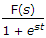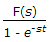# Electronics and Communication Engineering - Signals and Systems - Discussion

Discussion Forum : Signals and Systems - Section 1 (Q.No. 10)
10.
If £[f(t)] = F(s), then £[f(t - T)] =
est F(s)
e-st F(s)Explanation:

A linear system is one in which the relationship between input and output is linear.

All linear system possess the property of superposition i.e., if input u1 gives output y1 and input u2 gives output y2 then input u1 + u2 will give output y1 + y2.

Superposition implies homogencity i.e., if input u gives output y, then input ku gives output ky where k is a rational number.

In general a system is linear if a transformation T satisfies the relation

T(au1 + βu2) = aT(u1) + βT(u2)

where a and β are constant.

Discussion:
5 comments Page 1 of 1.

Kulwinder Kaur said:   4 years ago
Using time shifting property , the answer is;

f{t-k} = e^-ks F{s} //property.
f{t-T} = e^-sT F{s}.
(1)

Jyoti said:   5 years ago
Yes, right @Pratima.

Pratima Maiti said:   5 years ago
The answer should be e^-(sT) F(s).

Swapna said:   6 years ago
A digital signal processing system is described by the expression y(n)=2x(n) + x(n-1) +2y(n-1) than the system is,

Stable IIR Filter.
Astable IIR filter.

Can anyone tell me the correct answer for this?

Bipin Kumar said:   9 years ago
This can also be solved using time shifting property of Laplace.
(1)